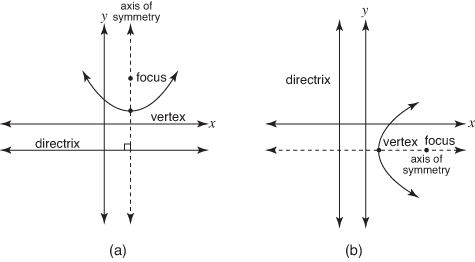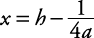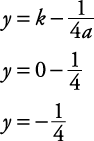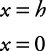## Parabola

A parabola is the set of points in a plane that are the same distance from a given point and a given line in that plane. The given point is called the focus, and the line is called the directrix. The midpoint of the perpendicular segment from the focus to the directrix is called the vertex of the parabola. The line that passes through the vertex and focus is called the axis of symmetry (see Figure 1.)

Figure 1. Two possible parabolas.The equation of a parabola can be written in two basic forms:

• Form 1: y = a( xh) 2 + k

• Form 2: x = a( yk) 2 + h

In Form 1, the parabola opens vertically. (It opens in the “ y” direction.) If a > 0, it opens upward. Refer to Figure 1(a). If a < 0, it opens downward. The distance from the vertex to the focus and from the vertex to the directrix line are the same. This distance isA parabola with its vertex at ( h, k), opening vertically, will have the following properties.

• The focus will be at.

• The directrix will have the equation.

• The axis of symmetry will have the equation x = h.

• Its form will be y = a( xh) 2 + k.

In Form 2, the parabola opens horizontally. (It opens in the “ x” direction.) If a > 0, it opens to the right. Refer to Figure 1(b). If a < 0, it opens to the left.

A parabola with its vertex at ( h, k), opening horizontally, will have the following properties.

• The focus will be at.

• The directrix will have the equation.

• The axis of symmetry will have the equation y = k.

• Its form will be x = a( yk) 2 + h.

##### Example 1

Draw the graph of y = x 2. State which direction the parabola opens and determine its vertex, focus, directrix, and axis of symmetry.

The equation y = x 2 can be written as

y = 1( x – 0) 2 + 0

so a = 1, h = 0, and k = 0. Since a > 0 and the parabola opens vertically, its direction is up (see Figure 2).

Vertex: ( h, k) = (0, 0)

Focus:.

Directrix:.

Axis of symmetry: